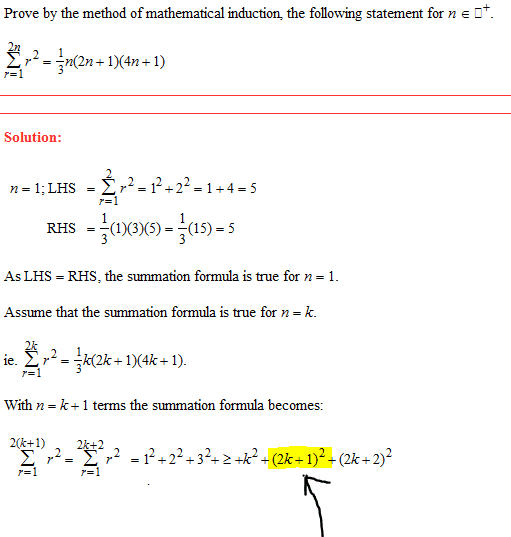# Series quick q.

phosphoShould the highlighted part not be (k+1)^2, could anyone explain?>

elvishatcher
Well, it makes sense to me that it would be (2k+1)^2. Since you're summing up to 2k+2, going backwards from the final term you would have (2k+2)^2 then (2k+2 - 1)^2 then (2k+2 - 2)^2, etc. which would make the second to last term (2k+1)^2 and not (k+1)^2. What doesn't make sense to me is that it shows the third to last term as k^2. Using the same method I just described, which I don't see a problem with, you get that the third to last term should be (2k+2 - 2)^2 = (2k)^2 = 4k^2. So, either there's a mistake or I'm missing something. If my reasoning is correct, though, it explains why it's (2k+1)^2

phospho
Well, it makes sense to me that it would be (2k+1)^2. Since you're summing up to 2k+2, going backwards from the final term you would have (2k+2)^2 then (2k+2 - 1)^2 then (2k+2 - 2)^2, etc. which would make the second to last term (2k+1)^2 and not (k+1)^2. What doesn't make sense to me is that it shows the third to last term as k^2. Using the same method I just described, which I don't see a problem with, you get that the third to last term should be (2k+2 - 2)^2 = (2k)^2 = 4k^2. So, either there's a mistake or I'm missing something. If my reasoning is correct, though, it explains why it's (2k+1)^2

That makes sense, thanks.

elvishatcher
Glad I could help - if you ever figure out some reason why I'm wrong and it should be k^2 not 4k^2, let me know